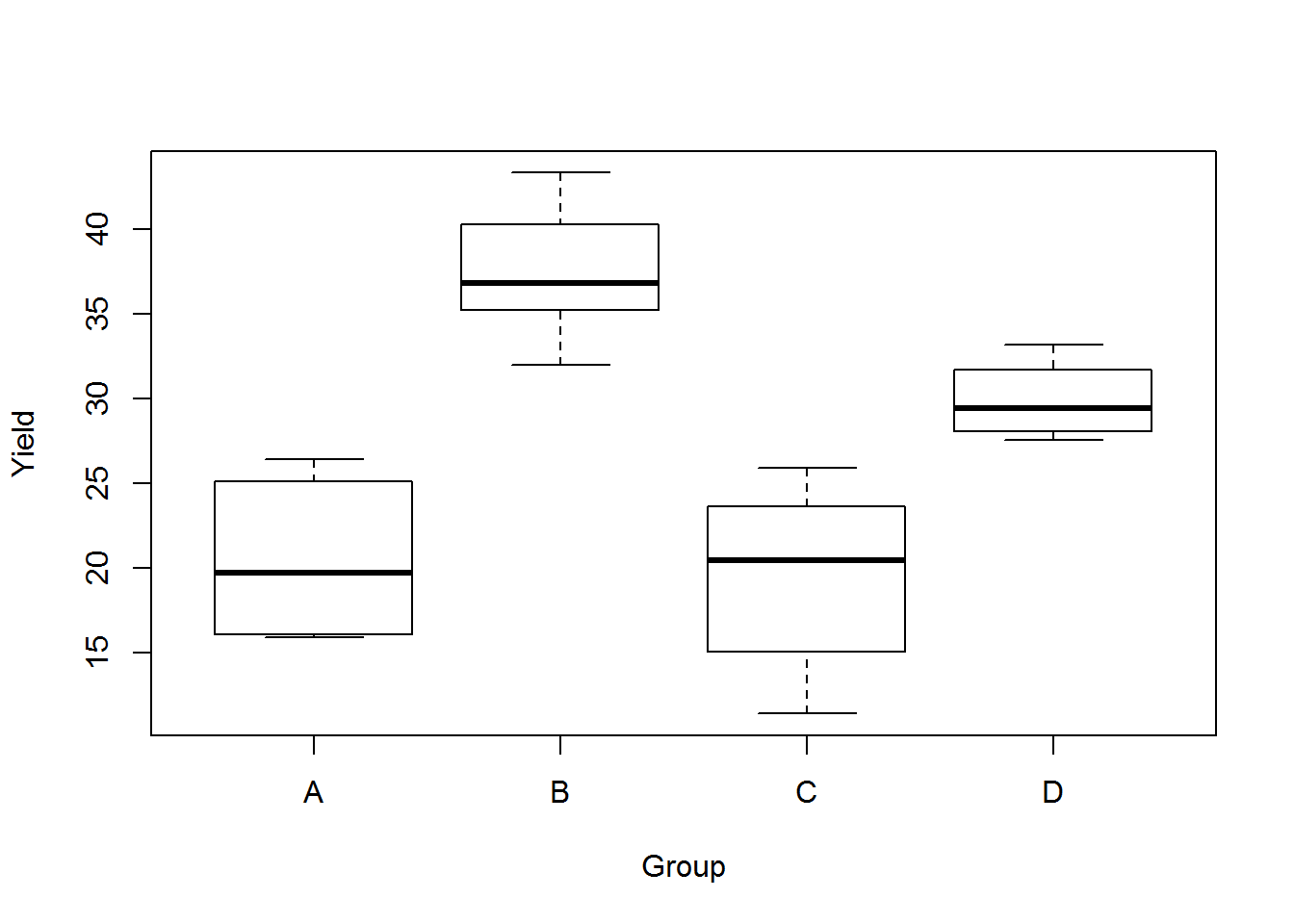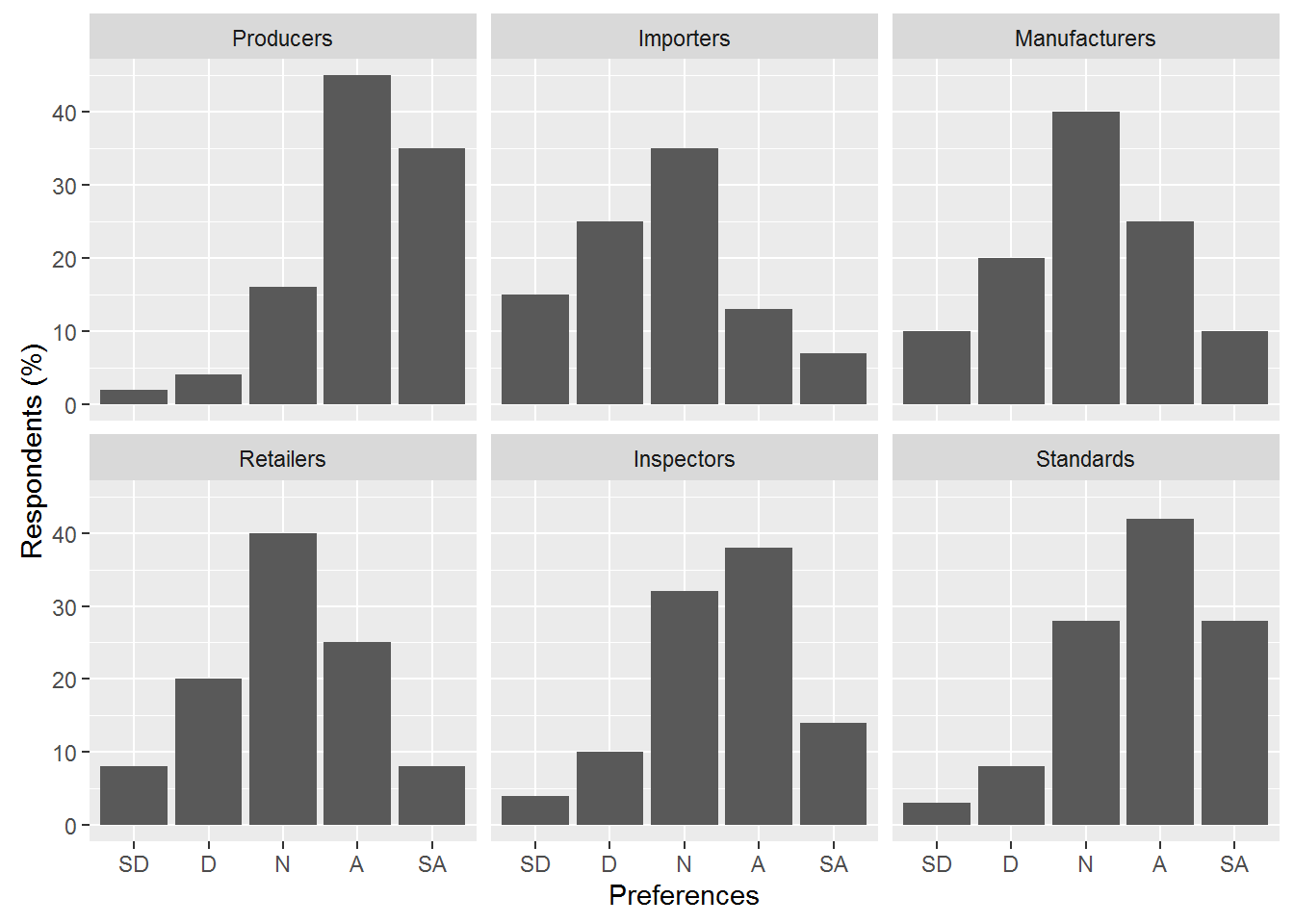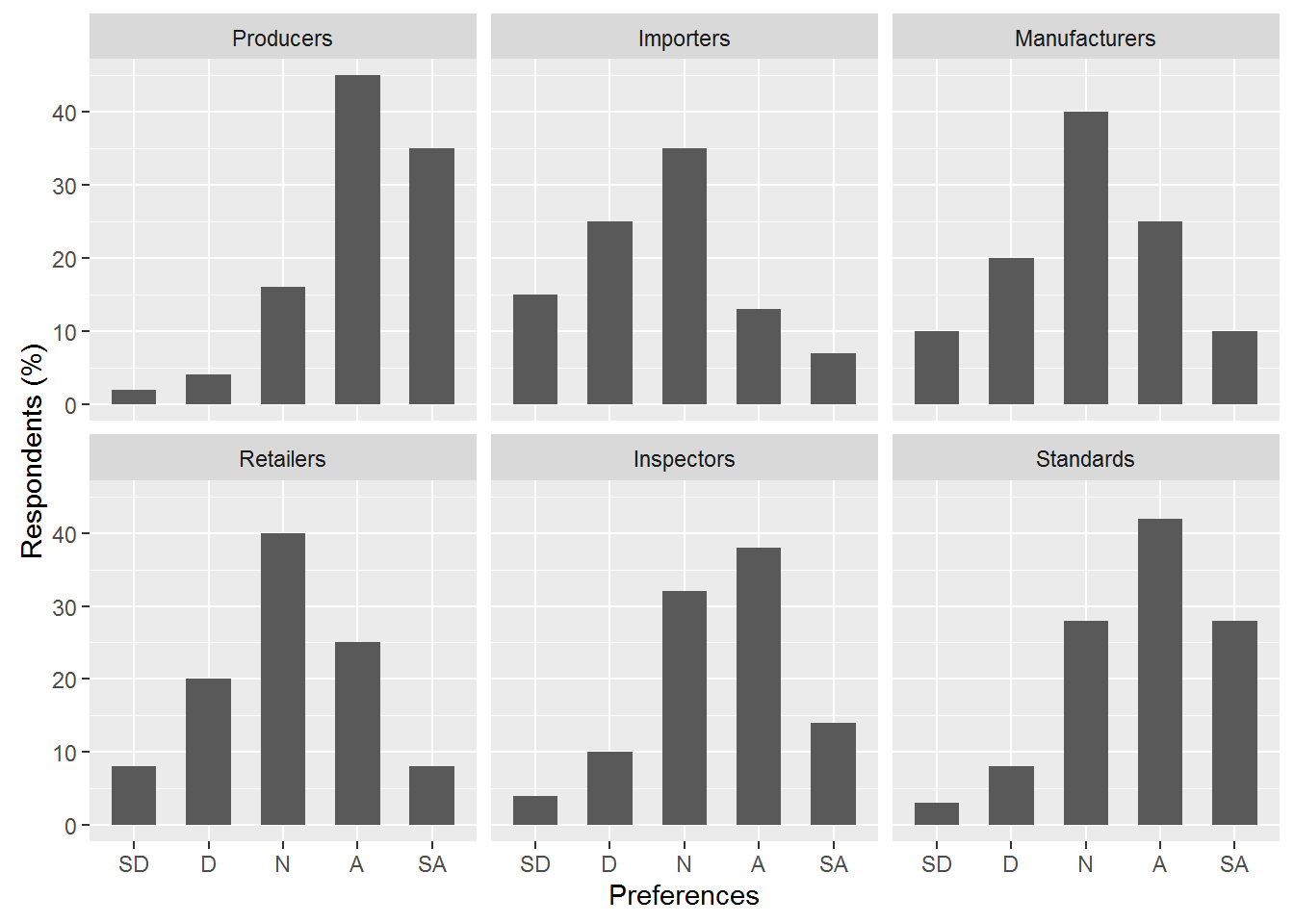# Module 6Top

## Objectives

At the and of this module you will have been demonstrated a good range of useful plots, with instructions to help understand the code. The grammar of graphics (ggplot) is very useful for a wide range of plots, and this module introduces some useful aspects. The page on Plotting gives some general comments on options that can be changed within the plots

## Contents

### 1. Looking at a simple one way completely randomised ANOVA design

(Melon yield data from Mead and Curnow One Way ANOVA)

``````melon <- read.csv("melon.csv")
# View(melon)

head(melon)  ## shows the top six lines of data``````
``````   Group Yield
1     A 25.12
2     A 17.25
3     A 26.42
4     A 16.08
5     A 22.15
6     A 15.92``````
``````#str(melon)   ## not run; gives the data structure
## Show there is a Factor in the data ``````

The data consists of two columns, one is a category (the group)

``names(melon)``
`` "Group" "Yield"``
``plot(melon)  ## Boxplot````````melon.mod1  <-  lm(Yield ~ Group, data=melon)  ## set up model

####   print coefficients from the model
melon.mod1``````
``````##
## Call:
## lm(formula = Yield ~ Group, data = melon)
##
## Coefficients:
## (Intercept)       GroupB       GroupC       GroupD
##      20.490       16.913       -1.007        9.407``````
``aov(melon.mod1)``
``````## Call:
##    aov(formula = melon.mod1)
##
## Terms:
##                     Group Residuals
## Sum of Squares  1292.2103  367.2364
## Deg. of Freedom         3        20
##
## Residual standard error: 4.285069
## Estimated effects may be unbalanced``````
``````summary(melon.mod1)
The output show the model, the summary of residuals and the coefficients of the given model as intercept and treatment effects. We generally require the means of the groups for reporting.

## Call:
## lm(formula = Yield ~ Group, data = melon)
##
## Residuals:
##     Min      1Q  Median      3Q     Max
## -8.0633 -2.5475 -0.5933  3.1633  6.4167
##
## Coefficients:
##             Estimate Std. Error t value Pr(>|t|)
## (Intercept)   20.490      1.749  11.713 2.08e-10 ***
## GroupB        16.913      2.474   6.836 1.21e-06 ***
## GroupC        -1.007      2.474  -0.407  0.68840
## GroupD         9.407      2.474   3.802  0.00112 **
## ---
## Signif. codes:  0 '***' 0.001 '**' 0.01 '*' 0.05 '.' 0.1 ' ' 1
##
## Residual standard error: 4.285 on 20 degrees of freedom
## Multiple R-squared:  0.7787, Adjusted R-squared:  0.7455
## F-statistic: 23.46 on 3 and 20 DF,  p-value: 9.316e-07``````
``replications(Yield ~ Group, data=melon)``

`## Group ## 6`

`A test for balance, we can ask for Replications:`

`!is.list(replications(Yield ~ Group, data=melon))`

`##  TRUE`

``````melon.aov  <-  aov(melon.mod1)  ## make a melon anova object
melon.aov``````
``````## Call:
##    aov(formula = melon.mod1)
##
## Terms:
##                     Group Residuals
## Sum of Squares  1292.2103  367.2364
## Deg. of Freedom         3        20
##
## Residual standard error: 4.285069
## Estimated effects may be unbalanced``````
``summary(melon.aov)``
``````##             Df Sum Sq Mean Sq F value   Pr(>F)
## Group        3 1292.2   430.7   23.46 9.32e-07 ***
## Residuals   20  367.2    18.4
## ---
## Signif. codes:  0 '***' 0.001 '**' 0.01 '*' 0.05 '.' 0.1 ' ' 1``````

We had previously checked that this design was balanced. We can also get the least significant difference using a function from the package agricolae

`````` \$statistics
##       Mean       CV  MSerror
##   26.81833 15.97813 18.36182
##
## \$parameters
##   Df ntr  t.value alpha       test name.t
##   20   4 2.085963  0.05 Fisher-LSD  Group
##
## \$means
##      Yield      std r      LCL      UCL   Min   Max
## A 20.49000 4.694422 6 16.84087 24.13913 15.92 26.42
## B 37.40333 3.950497 6 33.75421 41.05246 31.98 43.32
## C 19.48333 5.552551 6 15.83421 23.13246 11.42 25.90
## D 29.89667 2.229894 6 26.24754 33.54579 27.58 33.20
##
## \$comparison
##       Difference pvalue sig.        LCL        UCL
## A - B -16.913333 0.0000  *** -22.073978 -11.752689
## A - C   1.006667 0.6884       -4.153978   6.167311
## A - D  -9.406667 0.0011   ** -14.567311  -4.246022
## B - C  17.920000 0.0000  ***  12.759356  23.080644
## B - D   7.506667 0.0066   **   2.346022  12.667311
## C - D -10.413333 0.0004  *** -15.573978  -5.252689
##
## \$groups
## NULL``````
``````##       Difference pvalue sig.        LCL        UCL
## A - B -16.913333 0.0000  *** -22.073978 -11.752689
## A - C   1.006667 0.6884       -4.153978   6.167311
## A - D  -9.406667 0.0011   ** -14.567311  -4.246022
## B - C  17.920000 0.0000  ***  12.759356  23.080644
## B - D   7.506667 0.0066   **   2.346022  12.667311
## C - D -10.413333 0.0004  *** -15.573978  -5.252689``````
``````## Tables of means
## Grand mean
##
## 26.81833
##
##  Group
## Group
##     A     B     C     D
## 20.49 37.40 19.48 29.90
##
## Standard errors for differences of means
##         Group
##         2.474
replic.     6```

The Grand mean of the experiment can be obtained, and the means for the groups.
```
Tables of effects
Group
A      B      C      D
-6.328 10.585 -7.335  3.078``````

The following from the multicomp library gives the pairwise comparisons using Tukeys

``library(multcomp)``
``````TestPairsT <- glht(melon.aov, linfct = mcp(Group = "Tukey"))
summary(TestPairsT) # pairwise tests``````
``````##
##   Simultaneous Tests for General Linear Hypotheses
##
## Multiple Comparisons of Means: Tukey Contrasts##
## Fit: aov(formula = melon.mod1)
##
## Linear Hypotheses:
##            Estimate Std. Error t value Pr(>|t|)
## B - A == 0   16.913      2.474   6.836  < 0.001 ***
## C - A == 0   -1.007      2.474  -0.407  0.97662
## D - A == 0    9.407      2.474   3.802  0.00556 **
## C - B == 0  -17.920      2.474  -7.243  < 0.001 ***
## D - B == 0   -7.507      2.474  -3.034  0.03108 *
## D - C == 0   10.413      2.474   4.209  0.00224 **
## ---
## Signif. codes:  0 '***' 0.001 '**' 0.01 '*' 0.05 '.' 0.1 ' ' 1
## (Adjusted p values reported -- single-step method)

``````

This is a small example using ggplot2.  Labels are entered as lists, and then the counts in each of the Likert category as a dataframe, which uses the label lists too. It shows how to add data and plot 6 plots in a grid. The x axis is a Likert scale. (see Technical Asides for information about Likert scores). This would also work for an ordered categorical factor. BesT_Mod6_GGPLOT2bar

``library(ggplot2)``
``## Warning: package 'ggplot2' was built under R version 3.2.5``
``````typeBus <- c("Producers", "Importers", "Manufacturers", "Retailers",
"Inspectors", "Standards")

subcat <- c("SD", "D", "N", "A", "SA")

head(dat1 <- data.frame(sector =  gl(6, 5, length = 30, labels = typeBus),
Preferences = gl(5, 1, length = 30, labels = subcat),
perc = c(2, 4, 16, 45, 35, 15, 25, 35, 13, 7,
10, 20, 40, 25, 10, 8, 20,40, 25, 8,
4, 10, 32, 38, 14, 3, 8, 28, 42, 28)))``````
``````##      sector Preferences perc
## 1 Producers          SD    2
## 2 Producers           D    4
## 3 Producers           N   16
## 4 Producers           A   45
## 5 Producers          SA   35
## 6 Importers          SD   15``````
``````## bar plot

ggplot(dat1, aes(Preferences, perc)) + facet_wrap(~sector, nrow = 2) +
geom_bar(stat = "identity") + ylab("Respondents (%)")````````````## bar plot with thinner bars

ggplot(dat1, aes(Preferences, perc)) + facet_wrap(~sector, nrow = 2) +
geom_bar(stat = "identity", width = 0.6) + ylab("Respondents (%)")``````### Biplots

This site uses Akismet to reduce spam. Learn how your comment data is processed.